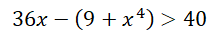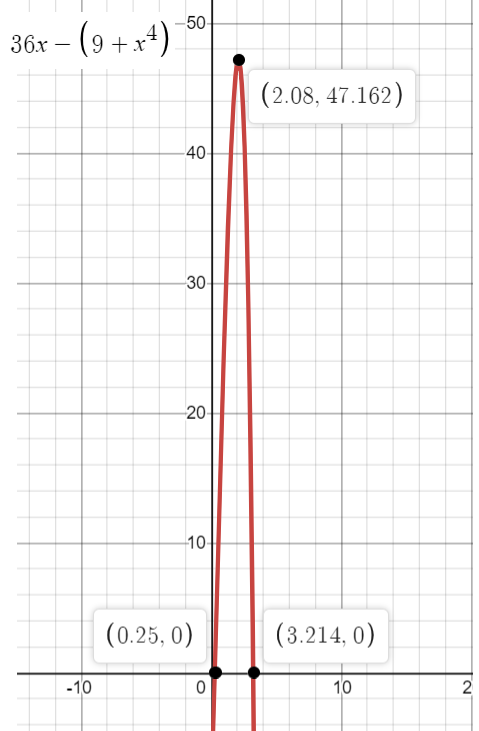# You are selling fidget spinners for \$32 each. If it costs you C(x)=9+x^4 dollars to produce x fidget spinners, is it possible to make more than \$40 in profit? Is it possible to have a loss of more than \$10,000,000,000?

Question
1 views

You are selling fidget spinners for \$32 each. If it costs you C(x)=9+x^4 dollars to produce x fidget spinners, is it possible to make more than \$40 in profit? Is it possible to have a loss of more than \$10,000,000,000?

check_circle

Step 1

We are selling fidget spinners for \$32 each.

We are C(x)=9+x^4 dollars to produce x fidget spinners.

We need to check whether it is possible to get more than \$40 in profit.

Summary:

selling price = 36x

production price = 9 + x4

Profit = selling price - production price

= 36x - (9 + x4)

Step 2

Profit will happen when profit > 0.

Here we need to check whether it is possible to get more than \$40 in profit.

In other words,.

Graphically we can see that,At x=2, profit = 47 > 40. Thus, it is possible to get more than \$40 in profit.

...

### Want to see the full answer?

See Solution

#### Want to see this answer and more?

Solutions are written by subject experts who are available 24/7. Questions are typically answered within 1 hour.*

See Solution
*Response times may vary by subject and question.
Tagged in